##### Question

In: Statistics and Probability

# A single die is rolled. Determine the probability of (show work) a) rolling a 6 given...

A single die is rolled. Determine the probability of (show work)

a) rolling a 6 given the number is even

b) rolling an even given the number is a 6

c) rolling a 5 given that the number is odd

d) rolling a 2 or a 6 given that the number is even

e) rolling a 4 given that the number is odd

## Solutions

##### Expert Solution

A single die is rolled.

Sample space = S = {1,2,3,4,5,6}

n(S) = 6

Let A be the event that number is even

A = {2,4,6}

n(A) = 3

Let B be the event that number is odd

B = {1,3,5}

n(B) = 3

a) rolling a 6 given the number is even

Let C be the event of rolling a 6

C ={6}

n(C) = 1

Required probability = P(C/A)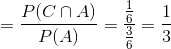b) rolling an even given the number is a 6

Required probability = P(A/C)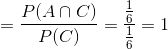c) rolling a 5 given that the number is odd

Let D be the event of rolling a 5

D = {5}

n(D) = 1

Required probability

= P(D/B)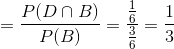d) rolling a 2 or a 6 given that the number is even

Let E be the event of rolling 2 or a 6

E = {2,6}

n(E) = 2

Required probability

= P(E/A)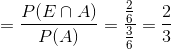e) rolling a 4 given that the number is odd

Let F be the event of rolling a 4

F = {4}

n(F) = 1

Required probability

= P(F/B)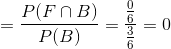## Related Solutions

##### Suppose a single fair die is rolled. Find the probability that it is a 2, given...
Suppose a single fair die is rolled. Find the probability that it is a 2, given that it is an odd number. The probability is
##### For the following exercises, assume two die are rolled. What is the probability of rolling a pair?
For the following exercises, assume two die are rolled.What is the probability of rolling a pair?
##### A die is rolled. Find the probability of the given event. (a) The number showing is...
A die is rolled. Find the probability of the given event. (a) The number showing is a 2; The probability is :       (b) The number showing is an even number; The probability is :      (c) The number showing is greater than 5; The probability is :
##### Rolling a Die If a die is rolled one time, find these probabilities: a. Getting a...
Rolling a Die If a die is rolled one time, find these probabilities: a. Getting a 7 b. Getting an odd number c. Getting a number less than 7 d. Getting a prime number (2, 3, or 5) 4.Rolling a Die If a die is rolled one time, find these probabilities: a. Getting a number greater than 0. b. Getting a number greater than or equal to 3 c. Getting a number greater than 2 and an even number d....
##### Suppose a 6-sided die and a 7-sided die are rolled. What is the probability of getting...
Suppose a 6-sided die and a 7-sided die are rolled. What is the probability of getting sum less than or equal to 5 for the first time on the 4th roll? Show your work to receive credit.
##### Two dice are rolled. Determine the probability of the following. Rolling an even number or a...
Two dice are rolled. Determine the probability of the following. Rolling an even number or a number greater than 10
##### What is the probability of rolling 6, 6 sided die and achieving a score of 18?...
What is the probability of rolling 6, 6 sided die and achieving a score of 18? (6 die, each one is 6 sided)
##### 24.Rolling Die Two dice are rolled. Find the probability of getting a.A sum of 8, 9,...
24.Rolling Die Two dice are rolled. Find the probability of getting a.A sum of 8, 9, or 10 b.Doubles or a sum of 7 c.A sum greater than 9 or less than 4 d.Based on the answers to a, b, and c, which is least likely to occur?
##### A fair red die and a fair green die are rolled. (a) What is the probability...
A fair red die and a fair green die are rolled. (a) What is the probability that the sum of the numbers is even? (b) What is the probability that the number on the red die is more than the number on the green die? (c) What is the probability that the number on the red die is twice the number on the green die? (d) What is the probability that the number on the red die is different from...
##### This question pertains to the probability of rolling a given total (adding the two die face-values)...
This question pertains to the probability of rolling a given total (adding the two die face-values) using 2 fair 6-sided dice. The possible results of rolling 2 fair 6-sided dice are the elements of the Cartesian product A × A, where A = {1, 2, 3, 4, 5, 6}. (a) How many elements does A × A have? (b) Partition the elements (x, y) of the set A × A according to the sum x + y. For example, (1,...